It is not surprising that people mix up formulas and functions in Excel. While both are used interchangeably, they are different. This guide compares Formulas to Functions in Excel to help you understand their differences and identify which is best to use for each case

## What is a Formula in Excel?

A formula is a mathematical equation from values or mathematical data in each cell. A can be used for calculating addition, subtraction, multiplication, or division of values, among several other operations.

Formulas in Excel are created by you, the user, depending on your goal or the calculation you want to make. It involves using operational signs and symbols and always begins with the equality sign.

### Example of Formula in Excel

Below are examples of formulas in Excel:

• =4+3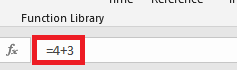• =A1+B1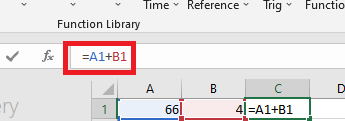• =4*3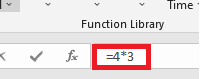• =A1/B1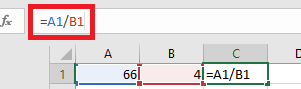The examples above include addition, multiplication, and division of cell values. The first and third examples perform operations on values, that is, 4 and 3, while the second and last operates on the cell value.

The equality sign is a requirement for writing formulas and does not influence the expected results.

Formulas in Excel may be simple or complex. The examples given above are simple and for simple calculations, while a more complex formula in Excel can be given as =B7+B8-(4*2)+1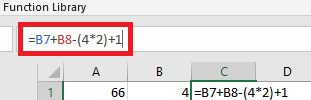## What is a Function in Excel?

A function is a type of formula that is pre-defined for performing specific operations. They allow users to perform advanced mathematical equations and also make simple calculations easy. Functions can also help you perform other tasks in Excel than mathematical calculations. For instance, you can count the number of cells in a spreadsheet, trim white spaces, substitute cell values, etc.

### Examples of Functions in Excel

The following are some examples of functions in  Excel:

• SUM
• AVERAGE
• COUNTIF
• COVARIANCE.P
• SUBTOTAL
• TOTAL

Like formulas, you must also begin a function line with the equality sign, but it does not influence the expected result of the operation. The general format for using a function in Excel is the equality sign, followed by the function name, and other syntaxes within a parenthesis. Here is an example:

=SUM(A1:A6)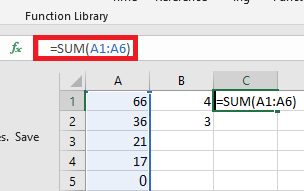Each function in Excel has a definition and cannot perform other operations other than its primary representation.

## What are the Differences Between a Formula and a Function in Excel?

Formulas and Functions in Excel are different, though a formula can contain one or more functions. The major difference between a formula and a function is that a formula is a string of signs or functions created by a user, while a function is predefined in the Excel background and it comes with the app.

Formulas are combinations of characters, and the characters can be mathematical signs or Excel functions, and a user can use them as it suits their goal. However, a user cannot customize or recreate a function. Here are other differences between a formula and a function in Excel:

• A function can be used within a formula, but a formula cannot be used within a function.
• Users can define their formula for any operation, but they must remember the exact syntax for using a function.
• Functions can make calculations over a wide range simple, while it would be complex when using formulas.

### Using Formula With Function in Excel

As mentioned earlier, you can use functions within a formula in Excel, especially for calculating complex mathematical or statistical problems. A formula for adding two cell values can be given as =A1+A2, while the function for performing the same operation is =SUM(A1:A2).

However, below is a formula that contains a function:

=A1+A2-(PRODUCT(A3:A4))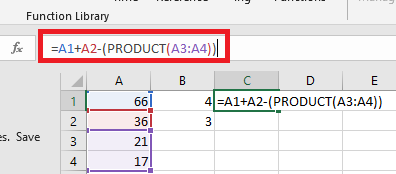The formula above sums the cell values of A1 and A2 and subtracts the product of A3 and A4 from the sum. Here PRODUCT is a function, but the whole expression is a formula.

### Conclusion

Functions in Excel are pre-defined syntaxes you can input into a formula, but formulas are user-defined and you can use them with or without a function.

You may also like to read:

How to Switch First and Last Names in Excel with Comma

How to Insert a Signature in Excel

How To Remove Empty Cells In Excel

How To Merge Tabs in Excel

How To Change Date Format In Excel To dd/mm/yyyy